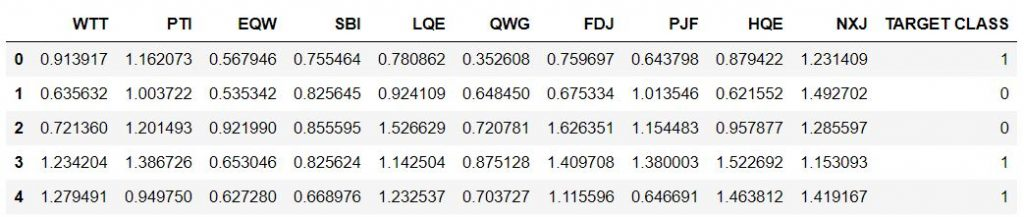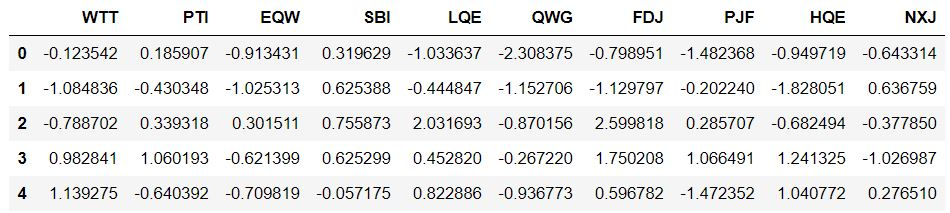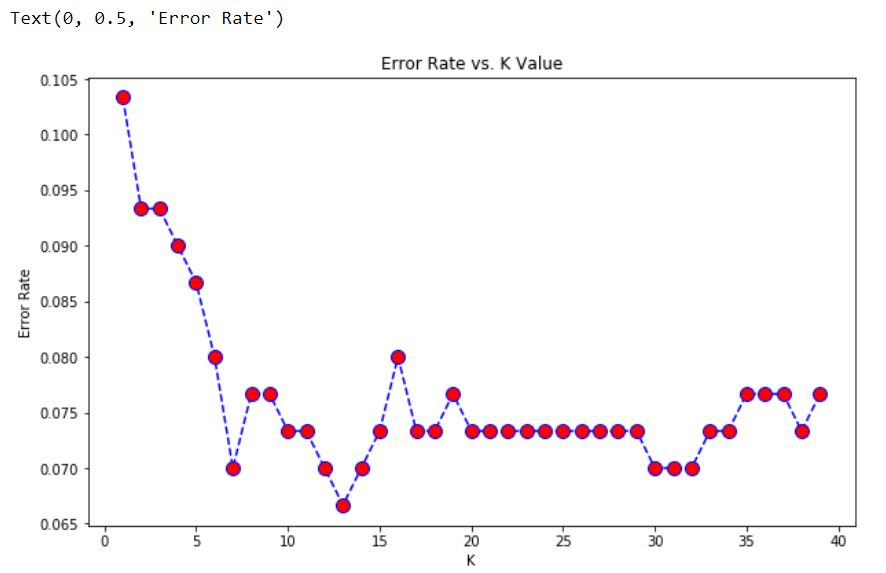# K Nearest Neighbors with Python | ML

How It Works ?
K-Nearest Neighbors is one of the most basic yet essential classification algorithms in Machine Learning. It belongs to the supervised learning domain and finds intense application in pattern recognition, data mining and intrusion detection.

The K-Nearest Neighbors (KNN) algorithm is a simple, easy-to-implement supervised machine learning algorithm that can be used to solve both classification and regression problems.

The KNN algorithm assumes that similar things exist in close proximity. In other words, similar things are near to each other. KNN captures the idea of similarity (sometimes called distance, proximity, or closeness) with some mathematics we might have learned in our childhood— calculating the distance between points on a graph. There are other ways of calculating distance, and one way might be preferable depending on the problem we are solving. However, the straight-line distance (also called the Euclidean distance) is a popular and familiar choice.

It is widely disposable in real-life scenarios since it is non-parametric, meaning, it does not make any underlying assumptions about the distribution of data (as opposed to other algorithms such as GMM, which assume a Gaussian distribution of the given data).

This article demonstrates an illustration of K-nearest neighbours on a sample random data using sklearn library.

Pre-requisites: Numpy, Pandas, matplotlib, sklearn

We’ve been given a random data set with one feature as the target classes. We’ll try to use KNN to create a model that directly predicts a class for a new data point based off of the features.

Import Libraries:

 `import` `pandas as pd ` `import` `seaborn as sns ` `import` `matplotlib.pyplot as plt ` `import` `numpy as np `

Let’s first visualize our data with Multiple Features.

Get the data:

Set index_col=0 to use the first column as the index.

 `df ``=` `pd.read_csv(``"Data"``, index_col ``=` `0``) ` ` `  `df.head() `

Output:Standardize the Variables:
Because the KNN classifier predicts the class of a given test observation by identifying the observations that are nearest to it, the scale of the variables matters. Any variables that are on a large scale will have a much larger effect on the distance between the observations, and hence on the KNN classifier than variables that are on a small scale.

 `from` `sklearn.preprocessing ``import` `StandardScaler ` ` `  `scaler ``=` `StandardScaler() ` ` `  `scaler.fit(df.drop(``'TARGET CLASS'``, axis ``=` `1``)) ` `scaled_features ``=` `scaler.transform(df.drop(``'TARGET CLASS'``, axis ``=` `1``)) ` ` `  `df_feat ``=` `pd.DataFrame(scaled_features, columns ``=` `df.columns[:``-``1``]) ` `df_feat.head() `

Output:Train Test Split Data and Use KNN model from sklearn library:

 `from` `sklearn.model_selection ``import` `train_test_split ` ` `  `X_train, X_test, y_train, y_test ``=` `train_test_split( ` `      ``scaled_features, df[``'TARGET CLASS'``], test_size ``=` `0.30``) ` ` `  `# Remember that we are trying to come up ` `# with a model to predict whether ` `# someone will TARGET CLASS or not. ` `# We'll start with k = 1. ` ` `  `from` `sklearn.neighbors ``import` `KNeighborsClassifier ` ` `  `knn ``=` `KNeighborsClassifier(n_neighbors ``=` `1``) ` ` `  `knn.fit(X_train, y_train) ` `pred ``=` `knn.predict(X_test) ` ` `  `# Predictions and Evaluations ` `# Let's evaluate our KNN model !  ` `from` `sklearn.metrics ``import` `classification_report, confusion_matrix ` `print``(confusion_matrix(y_test, pred)) ` ` `  `print``(classification_report(y_test, pred)) `

Output:

```[[133  16]
[ 15 136]]

precision    recall  f1-score   support

0       0.90      0.89      0.90       149
1       0.89      0.90      0.90       151

accuracy                           0.90       300
macro avg       0.90      0.90      0.90       300
weighted avg       0.90      0.90      0.90       300
```

Choosing a K Value:

Let’s go ahead and use the elbow method to pick a good K Value

 `error_rate ``=` `[] ` ` `  `# Will take some time ` `for` `i ``in` `range``(``1``, ``40``): ` `     `  `    ``knn ``=` `KNeighborsClassifier(n_neighbors ``=` `i) ` `    ``knn.fit(X_train, y_train) ` `    ``pred_i ``=` `knn.predict(X_test) ` `    ``error_rate.append(np.mean(pred_i !``=` `y_test)) ` ` `  `plt.figure(figsize ``=``(``10``, ``6``)) ` `plt.plot(``range``(``1``, ``40``), error_rate, color ``=``'blue'``, ` `                ``linestyle ``=``'dashed'``, marker ``=``'o'``, ` `         ``markerfacecolor ``=``'red'``, markersize ``=` `10``) ` ` `  `plt.title(``'Error Rate vs. K Value'``) ` `plt.xlabel(``'K'``) ` `plt.ylabel(``'Error Rate'``) `

Output:Here, we can see that that roughly after K>15 the error rate just tends to hover between 0.07-0.08 Let’s retrain the model with that and check the classification report.

 `# FIRST A QUICK COMPARISON TO OUR ORIGINAL K = 1 ` `knn ``=` `KNeighborsClassifier(n_neighbors ``=` `1``) ` ` `  `knn.fit(X_train, y_train) ` `pred ``=` `knn.predict(X_test) ` ` `  `print``(``'WITH K = 1'``) ` `print``(``'\n'``) ` `print``(confusion_matrix(y_test, pred)) ` `print``(``'\n'``) ` `print``(classification_report(y_test, pred)) ` ` `  ` `  `# NOW WITH K = 15 ` `knn ``=` `KNeighborsClassifier(n_neighbors ``=` `15``) ` ` `  `knn.fit(X_train, y_train) ` `pred ``=` `knn.predict(X_test) ` ` `  `print``(``'WITH K = 15'``) ` `print``(``'\n'``) ` `print``(confusion_matrix(y_test, pred)) ` `print``(``'\n'``) ` `print``(classification_report(y_test, pred)) `

Output:

```WITH K=1

[[133  16]
[ 15 136]]

precision    recall  f1-score   support

0       0.90      0.89      0.90       149
1       0.89      0.90      0.90       151

accuracy                           0.90       300
macro avg       0.90      0.90      0.90       300
weighted avg       0.90      0.90      0.90       300

WITH K=15

[[133  16]
[  6 145]]

precision    recall  f1-score   support

0       0.96      0.89      0.92       149
1       0.90      0.96      0.93       151

accuracy                           0.93       300
macro avg       0.93      0.93      0.93       300
weighted avg       0.93      0.93      0.93       300
```

Great! We were able to squeeze some more performance out of our model by tuning to a better K value.

Attention geek! Strengthen your foundations with the Python Programming Foundation Course and learn the basics.

To begin with, your interview preparations Enhance your Data Structures concepts with the Python DS Course.

My Personal Notes arrow_drop_upCheck out this Author's contributed articles.

If you like GeeksforGeeks and would like to contribute, you can also write an article using contribute.geeksforgeeks.org or mail your article to contribute@geeksforgeeks.org. See your article appearing on the GeeksforGeeks main page and help other Geeks.

Please Improve this article if you find anything incorrect by clicking on the "Improve Article" button below.

Article Tags :
Practice Tags :

1

Please write to us at contribute@geeksforgeeks.org to report any issue with the above content.